Example 27.2 Two-Way Random-Effects and Autoregressive Model for Panel Data

This example shows how you can use the SSM procedure to specify and fit the two-way random-effects model and the autoregressive model to analyze a panel of time series. These (and a few other) model types can also be fitted by the PANEL procedure, a SAS/ETS procedure that is specially designed to efficiently handle the cross-sectional time series data. However, because of the differences in their model fitting algorithms, generally the parameter estimates and other fit statistics produced by the SSM and PANEL procedures do not match. The SSM procedure always uses the (restricted) maximum likelihood for parameter estimation. The estimation method used by the PANEL procedure depends on the model type and the particular estimation options.

The cross-sectional data, Cigar, that are used in the section Getting Started: SSM Procedure are reused in this example. The output shown here is less extensive than the output shown in that section. The main emphasis of this example is how you can specify the two-way random effects model and the autoregressive model in the SSM procedure.

According to the two-way random effects model, the cigarette sales, lsales, can be described by the following equation: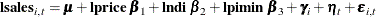This model represents lsales in regionand in yearas a sum of an overall intercept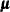, the regression effects due to lprice, lndi, and lpimin, a zero-mean, random effect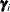associated with region, a zero-mean, random effect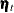associated with year, and the observation noise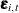. The region-specific random effectsand the year-specific random effectsare assumed to be independent, Gaussian sequences with variances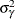and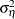, respectively. In addition, they are assumed to be independent of the observation noise, which is also assumed to be a sequence of independent, zero-mean, Gaussian variables with variance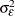.

You can specify and fit this model by using the following statements:

 proc ssm data=cigar;
id year interval=year;
parms s2g/ lower=(1.e-6);
array RegionArray{46} region1-region46;
do i=1 to 46;
RegionArray[i] = (region=i);
end;
/* region-specific random effects */
state gamma(46) T(I) cov1(I)=(s2g);
component regionEffect = gamma * (RegionArray);
/* year-specific random effect */
state eta(1) type=wn cov(D);
component timeEffect = eta;
irregular wn;
intercept = 1.0;
model lsales = intercept lprice lndi lpimin
timeEffect regionEffect wn;
run;


The PARMS statement defines s2g, a parameter that is restricted to be positive and is used later as the variance parameter for the region effect. Similarly the 46-dimensional array, RegionArray, of region-specific dummy variables is defined to be used later. The state subsection gamma corresponds to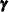, which is the 46-dimensional vector of region-specific, zero-mean, random effects. The component regionEffect extracts the proper element ofby using the array RegionArray. A constant column, intercept, is defined to be used later as an intercept term. The component timeEffect corresponds to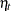, and wn specifies the observation noise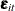. Finally the MODEL statement defines the model. Some of the tables that are produced by running these statements are shown in Output 27.2.1 through Output 27.2.5.

The model summary, shown in Output 27.2.1, shows that the model is defined by one MODEL statement, the dimension of the underlying state vector is 47 (becauseis 46-dimensional andis one-dimensional), the diffuse dimension is 4 (because of the four predictors in the model), and there are three parameters to be estimated.

Output 27.2.1: Two-Way Random-Effects Model: Model Summary

The SSM Procedure

Model Summary
Model Property Value
Number of Model Equations 1
State Dimension 47
Dimension of the Diffuse Initial Condition 4
Number of Parameters 3

Output 27.2.2 provides the likelihood information about the fitted model.

Output 27.2.2: Two-Way Random-Effects Model: Likelihood Summary

Likelihood Computation Summary
Statistic Value
Nonmissing Response Values Used 1380
Estimated Parameters 3
Initialized Diffuse State Elements 4
Normalized Residual Sum of Squares 1376
Full Log Likelihood 1459.03

Output 27.2.3 shows the regression estimates.

Output 27.2.3: Two-Way Random-Effects Model: Regression Estimates

Regression Parameter Estimates
Response Variable Regression Variable Estimate Standard Error t Value Pr > |t|
lsales intercept 2.798 0.1136 24.62 <.0001
lsales lprice -0.903 0.0365 -24.73 <.0001
lsales lndi 0.592 0.0246 24.08 <.0001
lsales lpimin 0.127 0.0398 3.18 0.0015

The ML estimate of s2g, a parameter specified in the PARMS statement, is shown in Output 27.2.4. It corresponds to, the variance of the region effect.

Output 27.2.4: Two-Way Random-Effects Model: Estimate ofEstimates of Named Parameters
Parameter Estimate Standard Error
s2g 0.0241 0.00512

Output 27.2.5: Variance Estimates ofand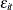Model Parameter Estimates
Component Type Parameter Estimate Standard Error
eta Disturbance Covariance Cov[1, 1] 0.000681 0.000264
wn Irregular Variance 0.005698 0.000224

The estimates of the other unknown parameters in the model are shown in Output 27.2.5. It shows the estimate of the variance of the irregular component wn and the estimate of the variance of the time effect.

The remainder of this example describes how you can specify and fit the following first-order vector autoregessive model to the cigarette data: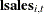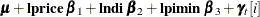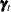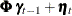This model represents lsales in regionand in yearas a sum of an overall intercept, the regression effects due to lprice, lndi, and lpimin, and theth element of a vector error term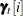. The multidimensional error sequenceis assumed to follow a first-order autoregression with a diagonal autoregressive coefficient matrix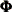and with a multivariate, white noise sequenceas its disturbance sequence. The covariance matrix of,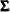, is assumed to be dense. Note that the dimension of the vectorsis the same as the number of cross-sections in the study (the number of regions in this example). Therefore, even for a relatively modest panel study, the total number of parameters to be estimated can get quite large. Therefore, in this example only the first three regions are considered in the analysis. The following statements specify and fit this model to the Cigar data set:

 proc ssm data=cigar;
where region <= 3;
id year interval=year;
array RegionArray{3} region1-region3;
do i=1 to 3;
RegionArray[i] = (region=i);
end;
state gamma(3) type=varma(p(d)=1) cov(g) print=(ar cov);
component eta = gamma*(RegionArray);
intercept = 1.0;
model lsales = intercept lprice lndi lpimin eta;
run;


The vectorsare specified in the STATE statement. The TYPE= specification signifies that the three-dimensional state subsection, gamma, follows a vector AR(1) model with a diagonal transition matrix and a disturbance covariance of a general form. The PRINT=(AR COV) option causes the SSM procedure to print the estimated AR coefficient matrix,, and the disturbance error covariance, respectively. The COMPONENT statement defines the appropriate error contribution (named eta),. Output 27.2.6 shows the estimated regression coefficients, Output 27.2.7 shows the estimate of, and Output 27.2.8 shows the estimate of:

Output 27.2.6: Autoregressive Model: Regression Estimates

The SSM Procedure

Regression Parameter Estimates
Response Variable Regression Variable Estimate Standard Error t Value Pr > |t|
lsales intercept 3.6857 0.3961 9.31 <.0001
lsales lprice -0.2356 0.0833 -2.83 0.0047
lsales lndi 0.1969 0.0774 2.54 0.0110
lsales lpimin 0.0737 0.0995 0.74 0.4588

Output 27.2.7: Estimate of the AR CoefficientAR Coefficient Matrix for gamma
Col1 Col2 Col3
Row1 0.925707 0 0
Row2 0 0.984015 0
Row3 0 0 0.960071

Output 27.2.8: Estimate of the Disturbance CovarianceDisturbance Covariance for gamma
Col1 Col2 Col3
Row1 0.000911 0.000342 0.000361
Row2 0.000342 0.002216 0.000172
Row3 0.000361 0.000172 0.000923